# Fourier integral

The non-discrete analogue of a Fourier series. The representation of a function given on a finite interval of the real axis by a Fourier series is very important. An analogous role is played by the representation of a functiongiven on the whole axis by a Fourier integral: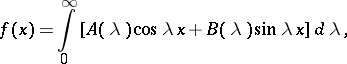(1)

where(2)The representation (1) can be constructed formally by assuming the existence of the described integrals. It is valid, for example, for a smooth functionof compact support. There are a great number of tests guaranteeing equation (1) in some sense or other. The substitution of (2) into (1) gives the so-called Fourier integral formula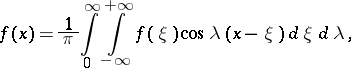(3)

whose proof reduces to the tests mentioned above. It can be of great advantage to use the representation ofby the simple Fourier integral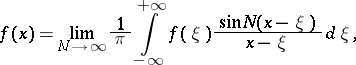which is obtained from (3) by writing the inner integral as a limit over the interval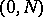and changing the order of integration. In applied sciences the representation (1) is often interpreted as expansion into harmonics: Ifthen (1) takes the form: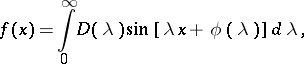and thusis represented by a superposition of harmonics with frequencieswhich continuously fill the real semi-axis, while the amplitudeand the initial phasedepend on.

In many cases (in particular for complex-valued functions) it is more convenient to present (1) in exponential form:(4)

where(5)

The function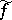is called the Fourier transform of(in applied sciences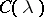is called the frequency characteristic or the spectrum of).

Under the condition that the functionis summable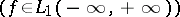, the functionis bounded, uniformly continuous on the real axis andas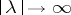. The functionneed not be integrable and so the integral (4) need not exist. However, (4) admits a reasonable interpretation if methods of summing integrals are used (here one can consider not only pointwise convergence but also convergence in the mean). For example, the arithmetical means of the truncated Fourier integrals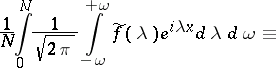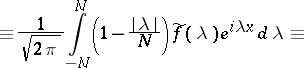of a summable functionconverge in the mean toalmost-everywhere as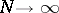. With additional restrictions onone can obtain more specific assertions. For example, ifand has bounded variation in a neighbourhood of, then(6)

In applications one often uses the representation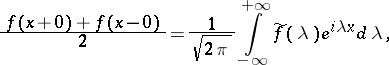which is true for an absolutely-integrable functionthat is piecewise smooth in every finite interval, where the integral on the right-hand side is taken to mean the principal value (6). The Fourier integral is also studied whenis assumed to be locally summable and with some hypotheses imposing restrictions on the behaviour ofat. For example, let,, then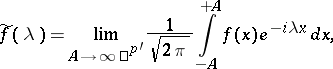(7)

where the limit is understood to be convergence in the mean of order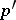,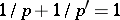(however, the limit in (7) also exists in the sense of convergence almost-everywhere). This result acquires a simple form when(see Plancherel theorem).

The theory of multiple Fourier integrals is constructed analogously when one discusses the expansion of a function given on an-dimensional space. The concept of the Fourier integral has been extended also to generalized functions.